Скачать презентацию Since a triangle has three sides it has

3d50181a04863141cbf094eab53a96af.ppt

• Количество слайдов: 31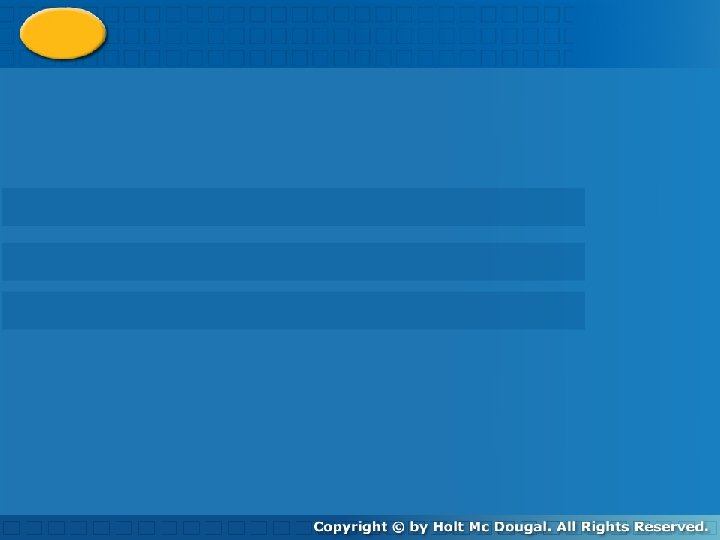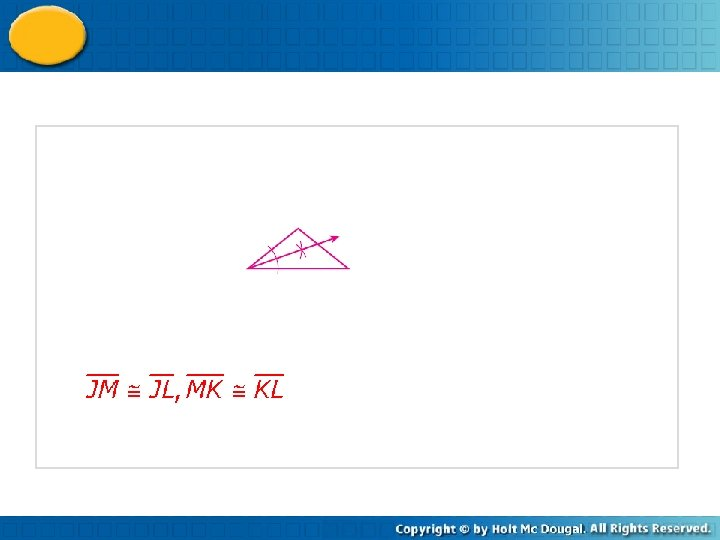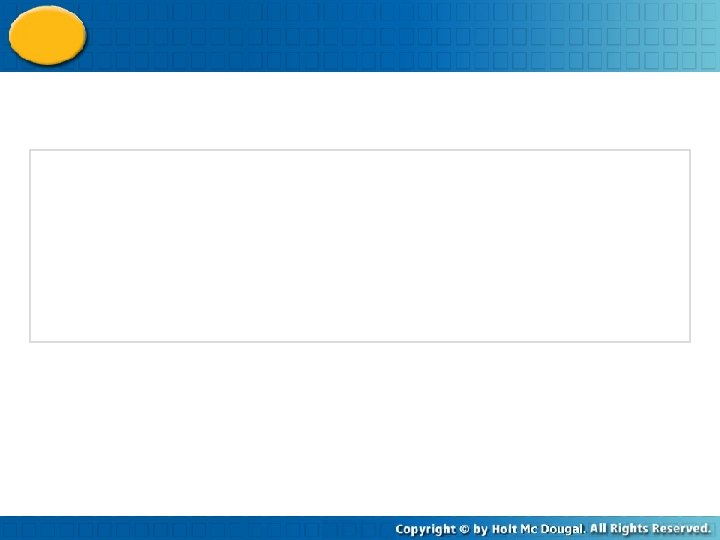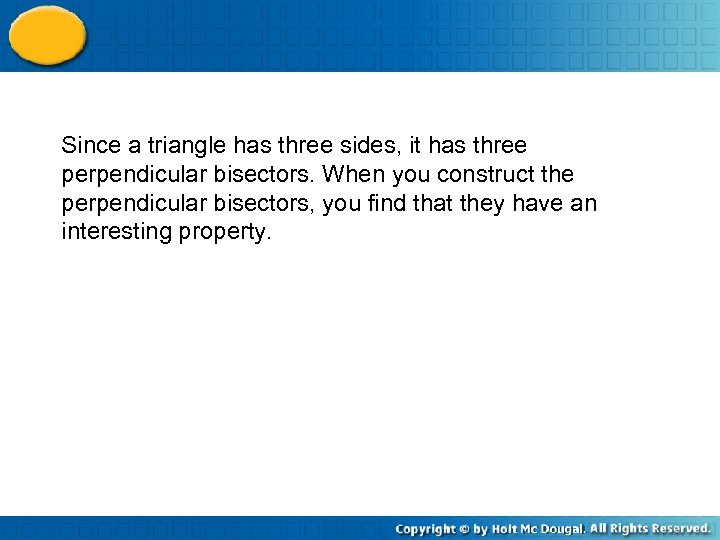Since a triangle has three sides, it has three perpendicular bisectors. When you construct the perpendicular bisectors, you find that they have an interesting property.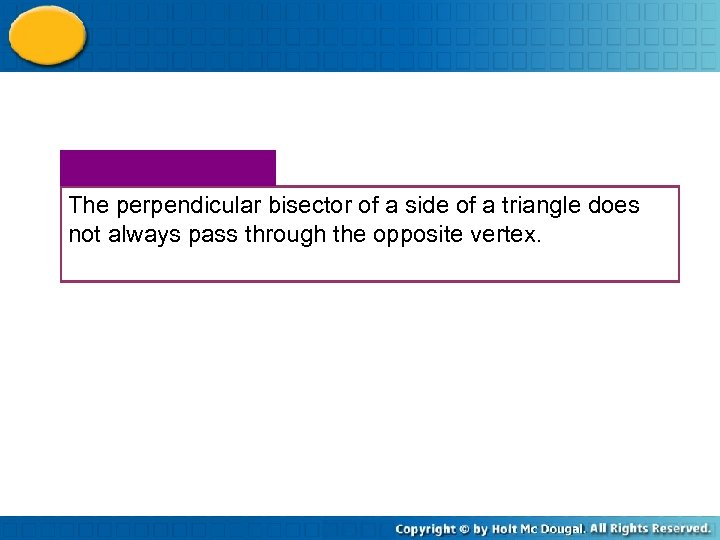The perpendicular bisector of a side of a triangle does not always pass through the opposite vertex.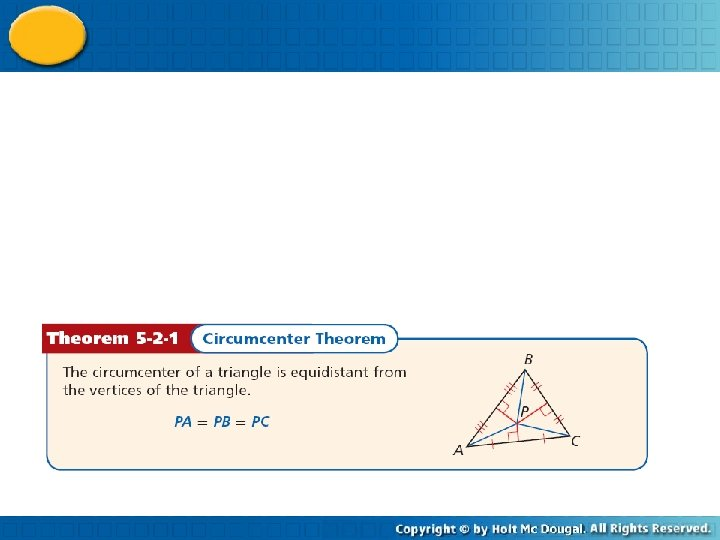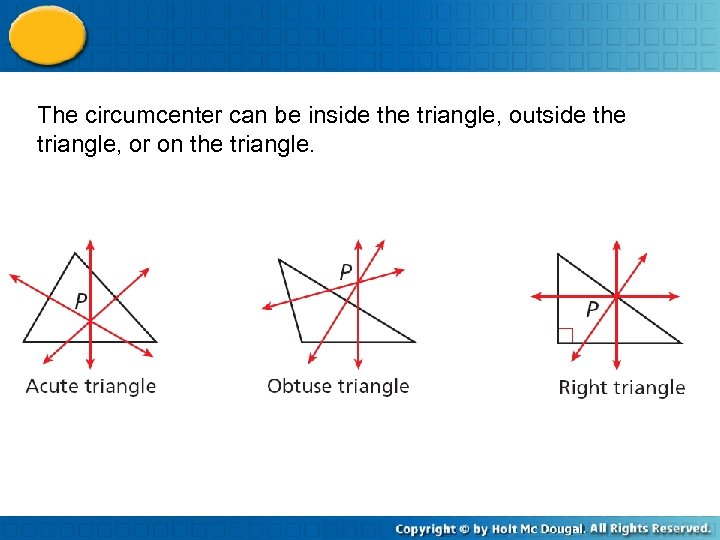The circumcenter can be inside the triangle, outside the triangle, or on the triangle.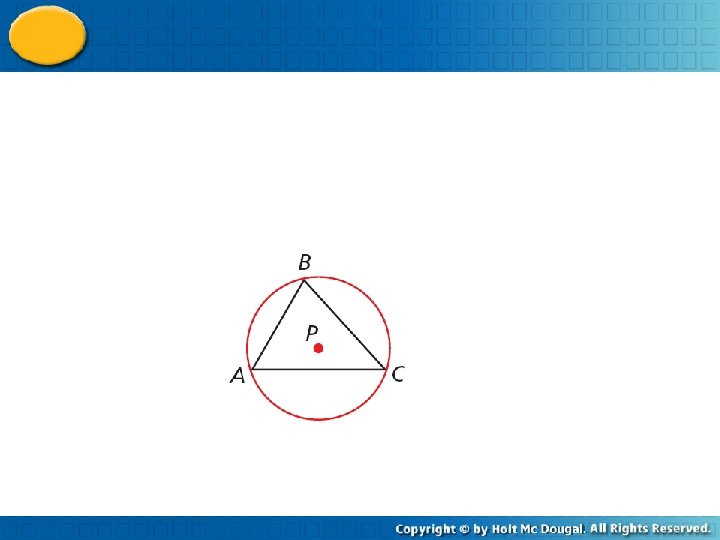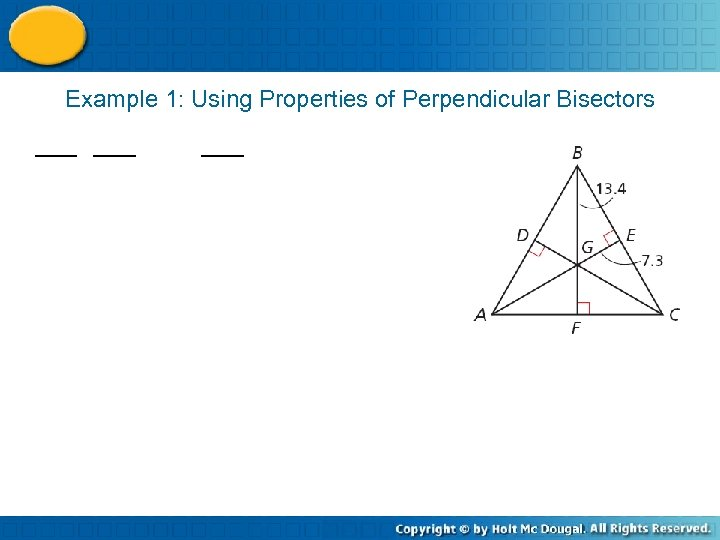Example 1: Using Properties of Perpendicular Bisectors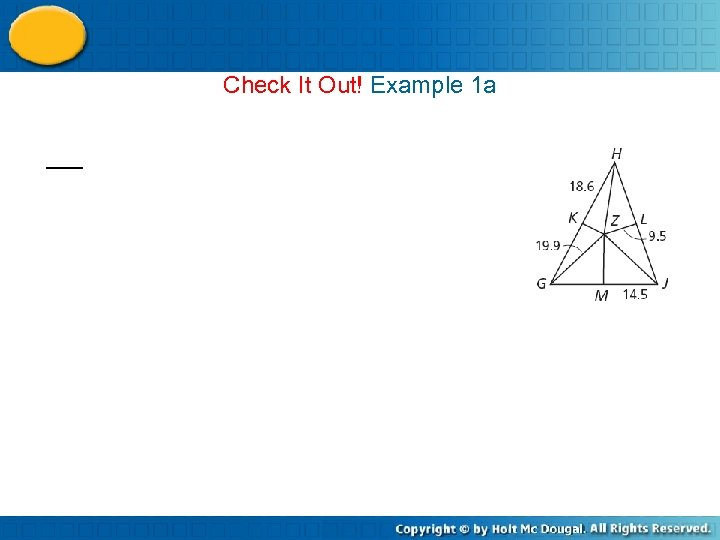Check It Out! Example 1 a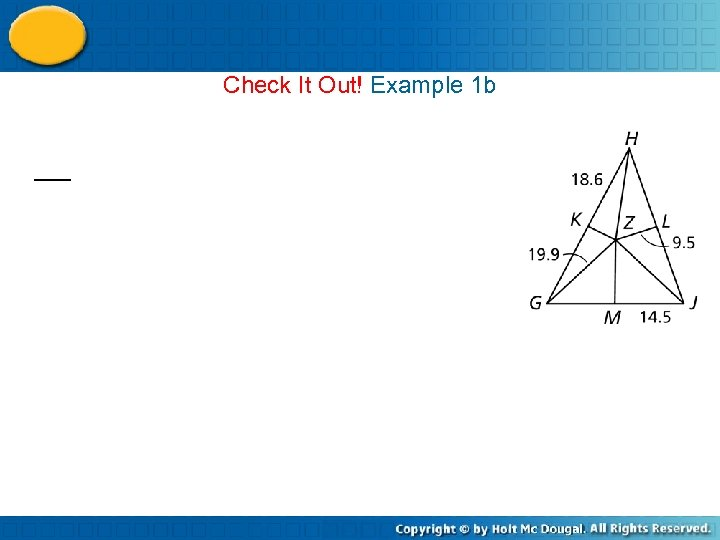Check It Out! Example 1 b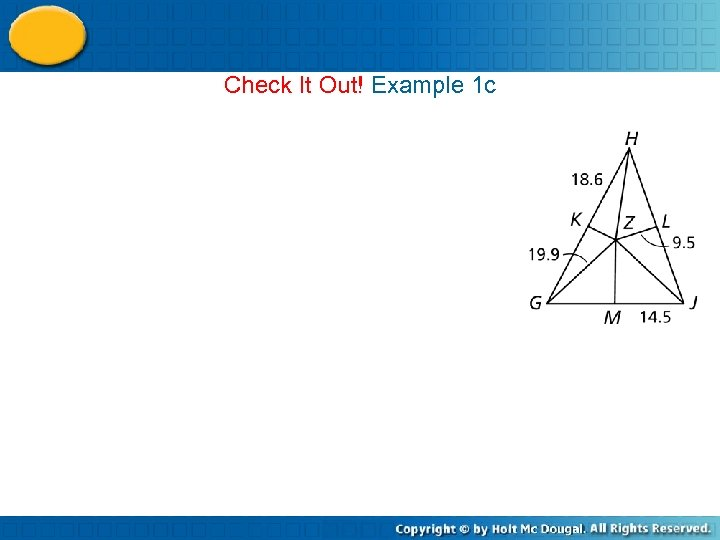Check It Out! Example 1 c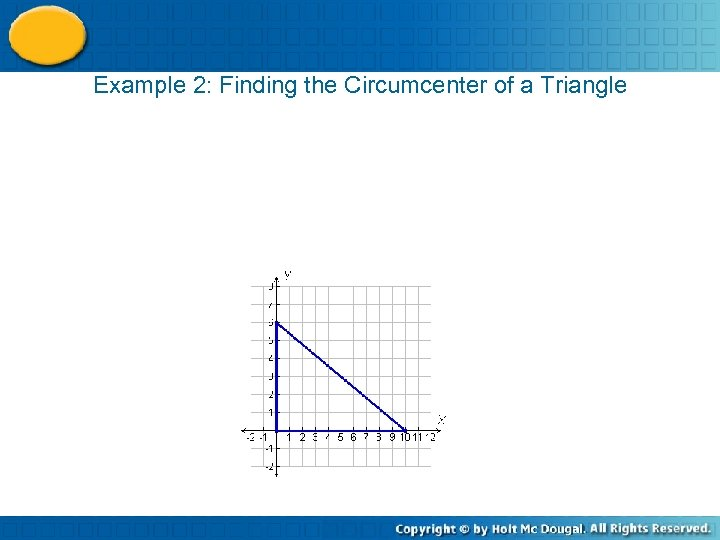Example 2: Finding the Circumcenter of a Triangle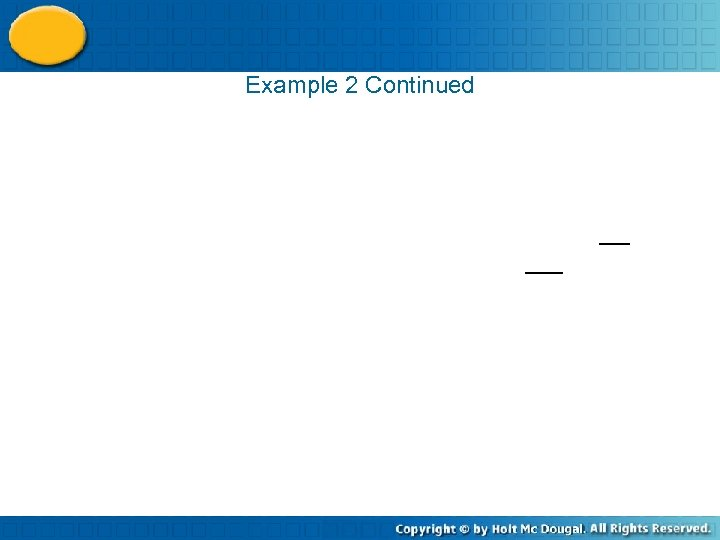Example 2 Continued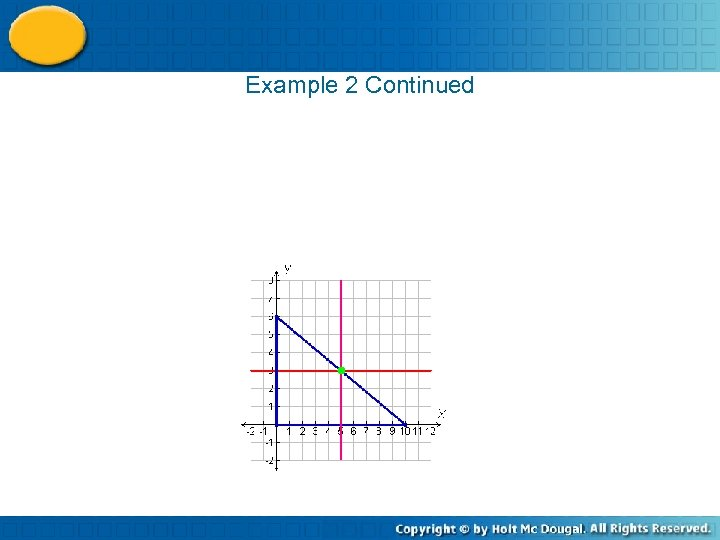Example 2 Continued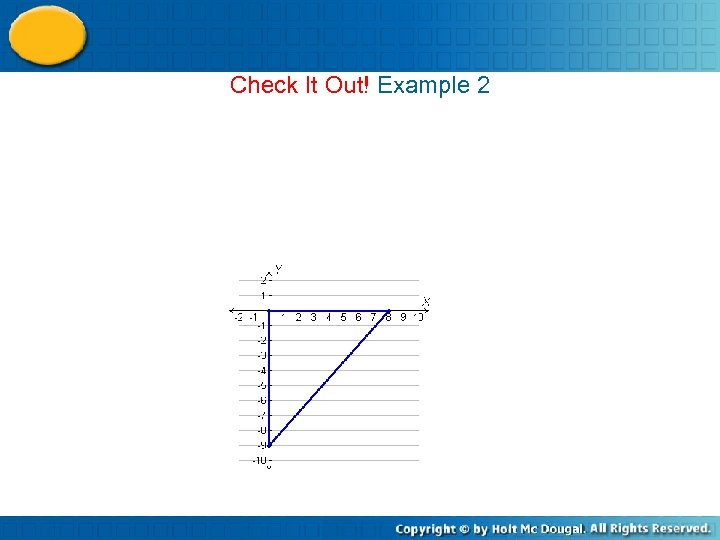Check It Out! Example 2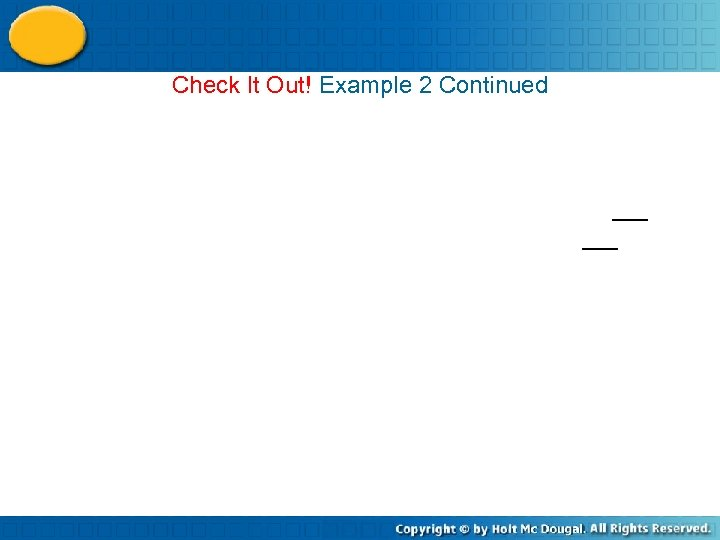Check It Out! Example 2 Continued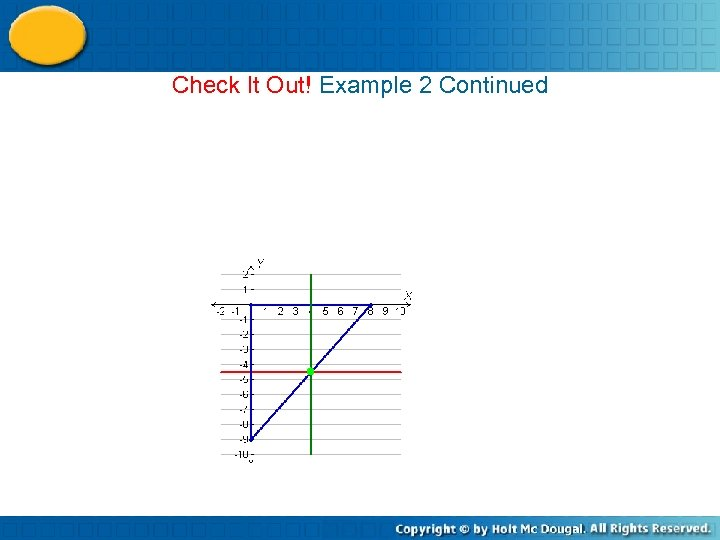Check It Out! Example 2 Continued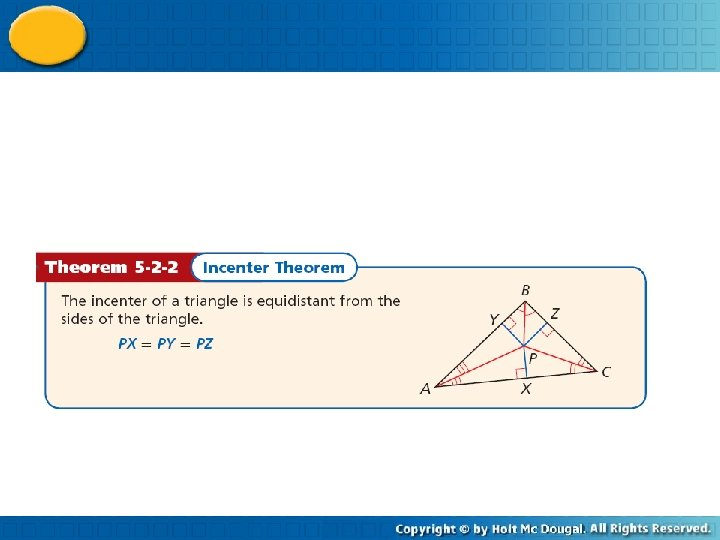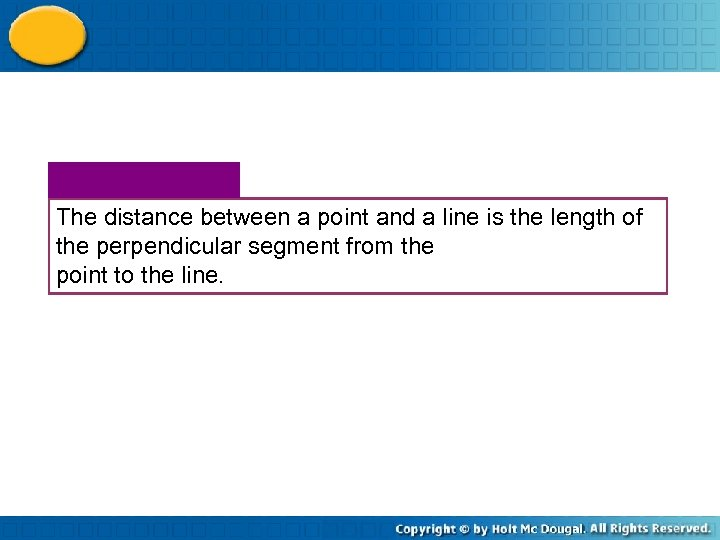The distance between a point and a line is the length of the perpendicular segment from the point to the line.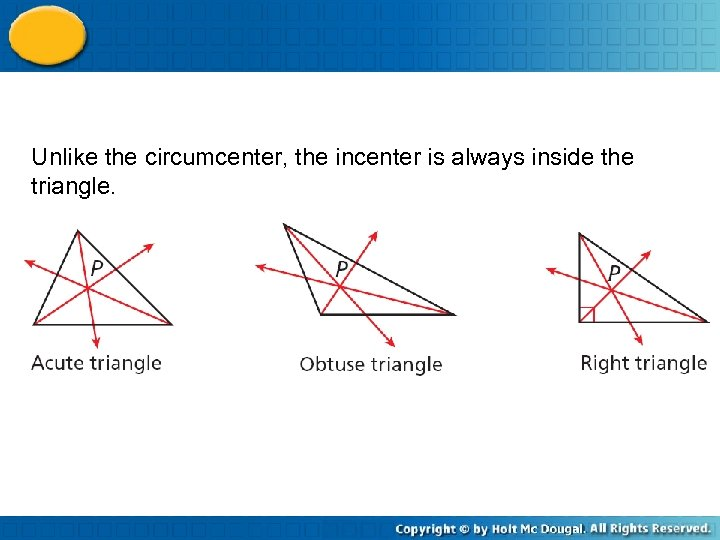Unlike the circumcenter, the incenter is always inside the triangle.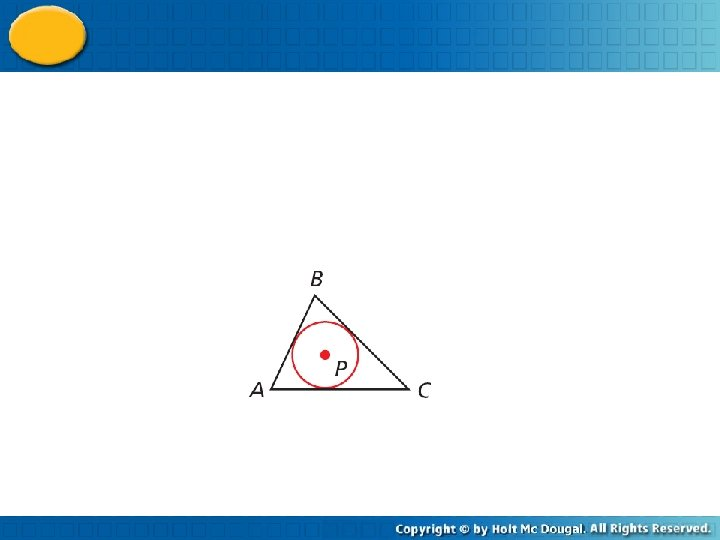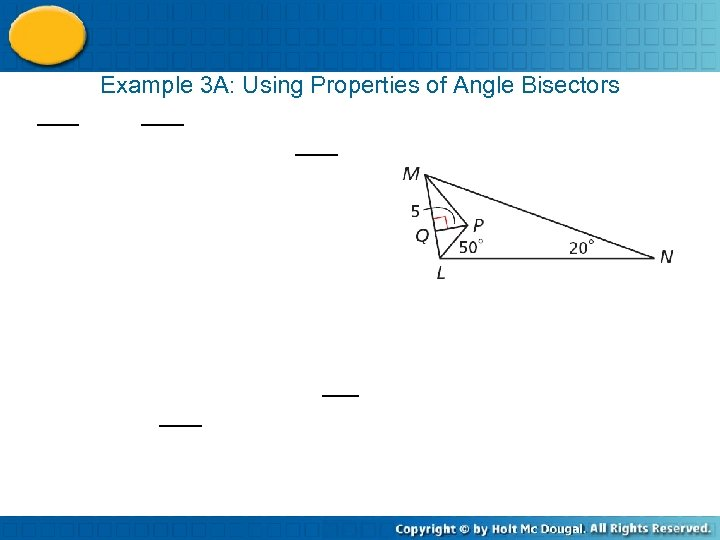Example 3 A: Using Properties of Angle Bisectors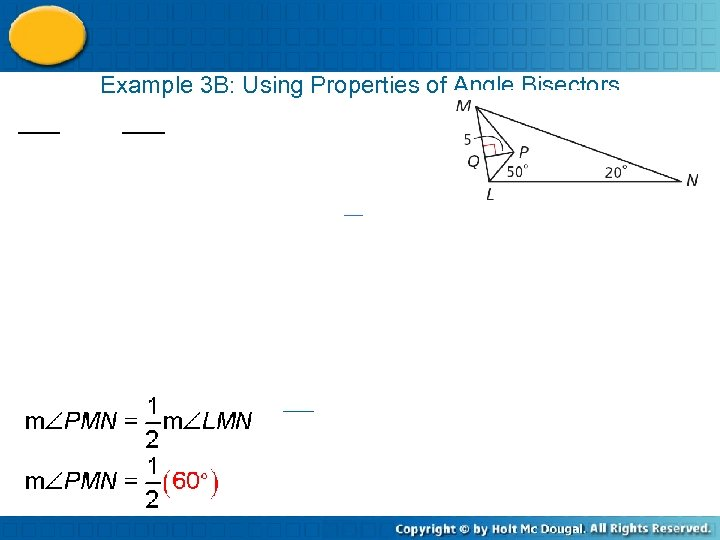Example 3 B: Using Properties of Angle Bisectors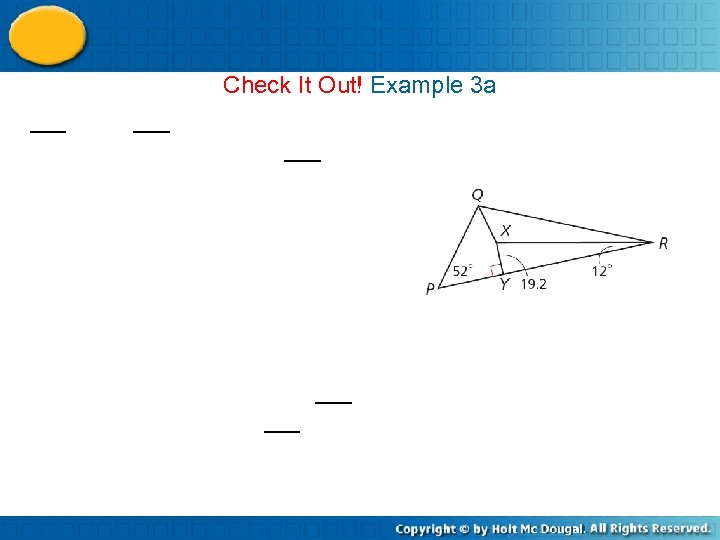Check It Out! Example 3 a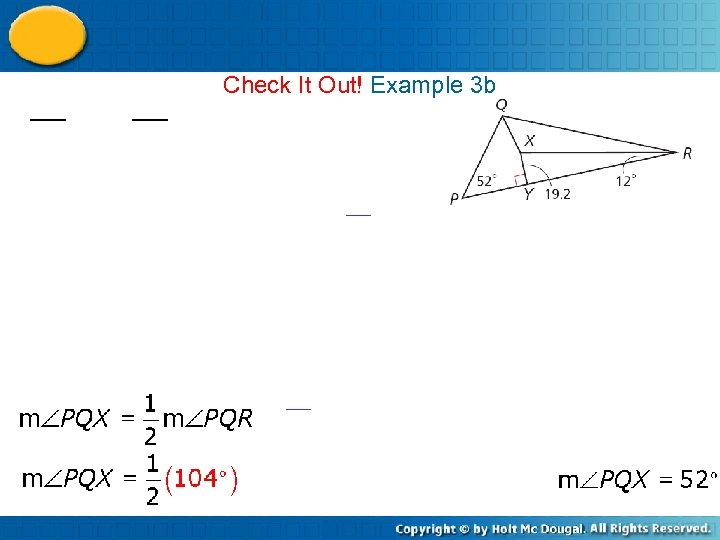Check It Out! Example 3 b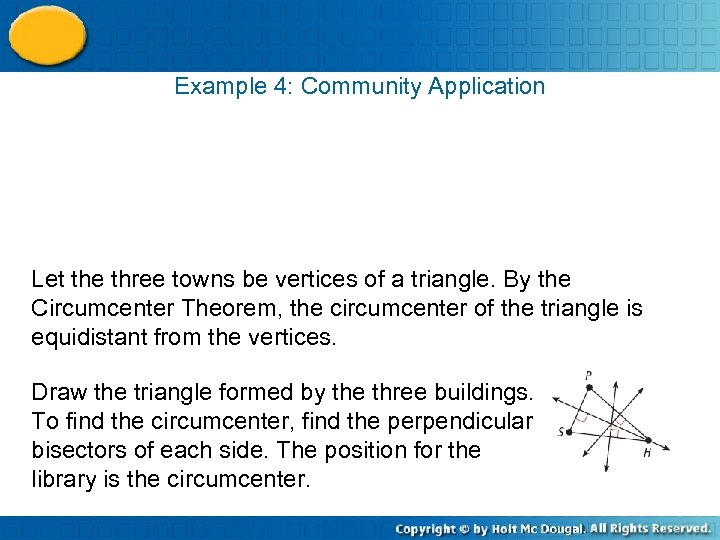Example 4: Community Application Let the three towns be vertices of a triangle. By the Circumcenter Theorem, the circumcenter of the triangle is equidistant from the vertices. Draw the triangle formed by the three buildings. To find the circumcenter, find the perpendicular bisectors of each side. The position for the library is the circumcenter.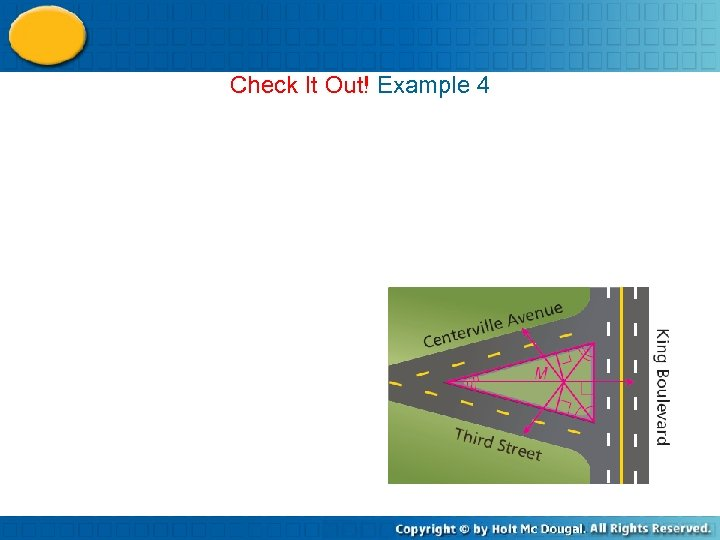Check It Out! Example 4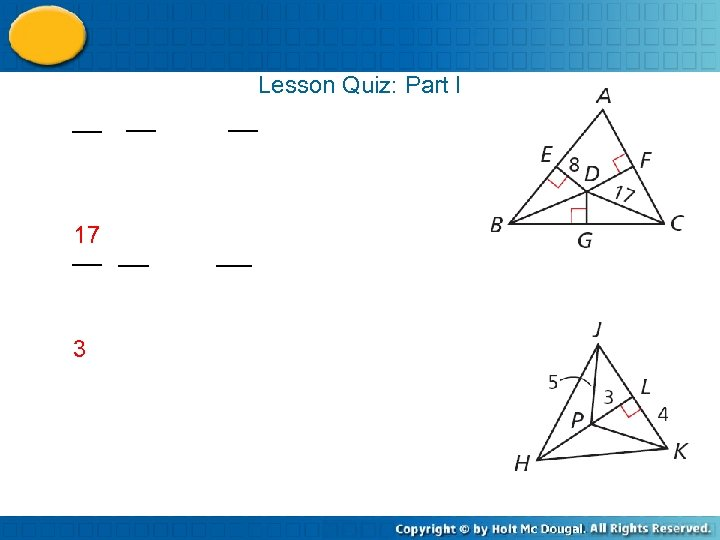Lesson Quiz: Part I 17 3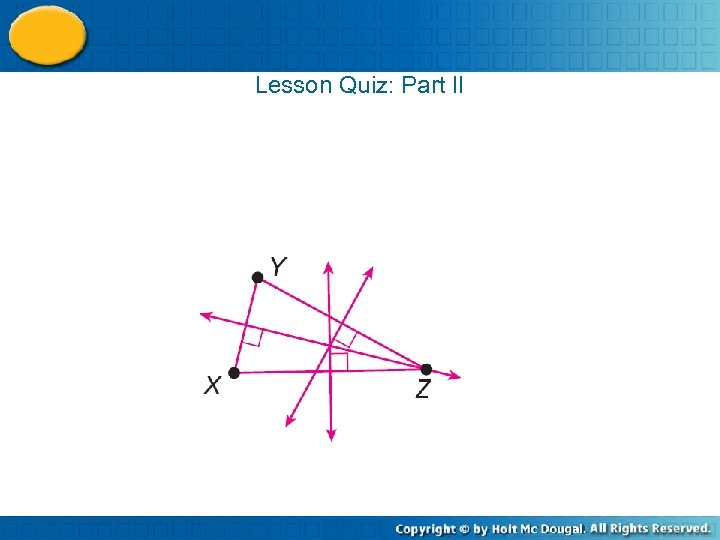Lesson Quiz: Part II